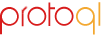A library designed for rapid assembly, querying, and interactive visual rendering of diagrams and common abstract mathematical structures.

## Purpose

This library makes it possible to easily introduce specific kinds of interactive graph diagrams into HTML documents. It depends primarily on D3.js; it also supports initialization of jQuery-wrapped document elements as interactive diagrams.

## Repository and Packages

The official repository is maintained on GitHub. The JavaScript package is also available on npm and the Python package is available on PyPI.
``````
npm install protoql
pip install protoql
``````
The latest version of the JavaScript library is also hosted at `http://protoql.org/lib/protoql.js`, although we recommend maintaining a copy on your own server at this time.

## Example

Using the library is straightforward. Simply include a diagram description in document elements, and then apply the visualization constructor to those elements.
``````
<script src="//d3js.org/d3.v3.min.js"></script>
<script src="//code.jquery.com/jquery-2.2.0.min.js"></script>
<script src="//protoql.org/lib/protoql.js"></script>
<div class="pql">
table([
[["dd`r``lorem`ipsum`dolor`sit amet"], ("s`r:lorem``consectetur"), ["dl:tempor``sed do`eiusmod tempor"]],
[null, ["dl``incididunt`ut labore"], null],
])
</div>
</body>
``````
The diagram above would render as shown below.
table([ [["dd`r``lorem`ipsum`dolor`sit amet"], ("s`r:lorem``consectetur"), ["dl:tempor``sed do`eiusmod tempor"]], [null, ["dl``incididunt`ut labore"], null], [("r:dolor``lorem`ipsum`dolor`sit amet"), ("r`uu``incididunt"), ["consectetur`adipiscing`elit"]] ])
The notation for tables being used above (inspired by the LaTeX xy package) is as follows:
• all nodes are strings describing the edges originating at that node and the node label, where:
• each individual arrow description string is of the form `ddll` (this is an example) and indicate the direction the arrow travel in the grid (e.g., `d` for "down"),
• the individual arrow strings are separated using a backquote character (i.e., ```),
• the arrow descriptions and the label are separated using a double backquote (i.e., ````),
• the backquote character is also the newline separator for the label string, and
• circular nodes are delimited using `(`...`)`, rectangular nodes are delimited using `[`...`]`, and empty space is denoted using `null`;
• each row is represented using a list of node entries;
• the table is represented as a list of rows.
Supported diagram notations include `table`, `relation`, and `graph`. For example, the following will create a diagram with a small graph containing five nodes and three edges.
``````
relation({'a','b','c'}, {'x','y'}, {('a','x'),('b','y'),('a','y')})
``````
relation({'a','b','c'}, {'x','y'}, {('a','x'),('b','y'),('a','y')})
When using `relation`, it is possible to supply one set or two sets before supplying the edge set. In either case, two sets will be arranged side-by-side (two copies of the same set, or the two distinct sets, going from left to right), and the edges will be added between the two sets. When using `graph`, the nodes will be arranged from top to bottom, left to right as they are listed (roughly accommodating the dimensions of the diagram).
``````
graph({'a','b','c','d','e'}, {('a','b'),('b','c'),('a','a'),('c','b')})
``````
graph({'a','b','c','d','e'}, {('a','b'),('b','c'),('a','a'),('c','b')})
The set is optional in both `relation` and `graph` diagrams (if it is not supplied, it will be derived from the edge set). Adding the `!` decorator fixes the diagram (zooming, panning, and dragging are disabled); this works for all three diagram types.
``````
!graph({('a','b'),('b','c'),('a','a'),('c','b')})
``````
!graph({('a','b'),('b','c'),('a','a'),('c','b')})
By using the `#` decorator, it is possible to create a diagram with a textbox allowing the user to edit the data from which the diagram is generated. Double-clicking any empty area in the diagram rebuilds the diagram using the data that currently appears in the textbox.
``````
#graph({('a','b'),('b','c'),('a','a'),('c','b')})
``````
#graph({('a','b'),('b','c'),('a','a'),('c','b')})